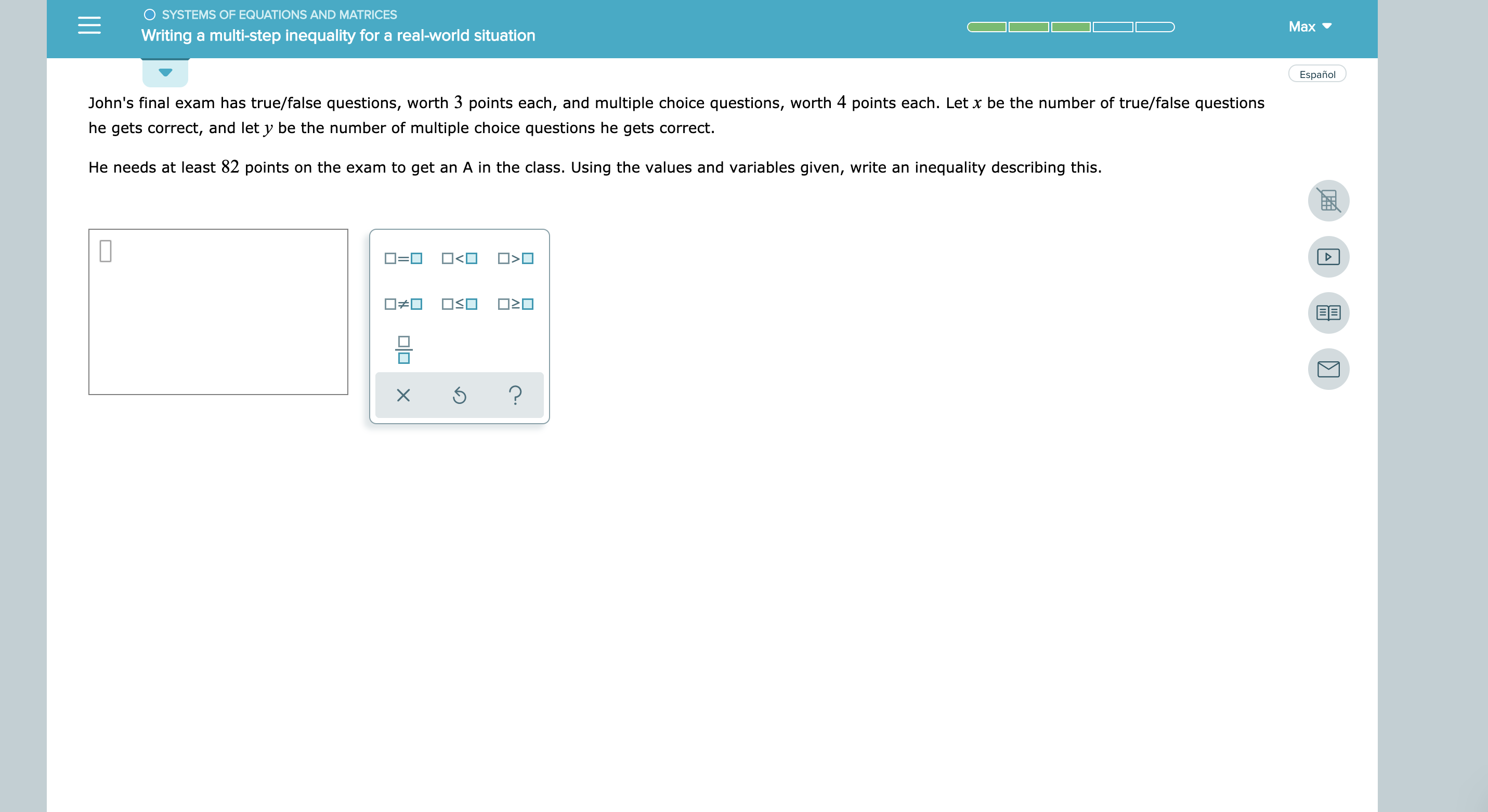# O SYSTEMS OF EQUATIONS AND MATRICES Max Writing a multi-step inequality for a real-world situation Español John's final exam has true/false questions, worth 3 points each, and multiple choice questions, worth 4 points each. Let x be the number of true/false questions he gets correct, and let y be the number of multiple choice questions he gets correct He needs at least 82 points on the exam to get an A in the class. Using the values and variables given, write an inequality describing this. =

Question

see attachmenthelp_outlineImage TranscriptioncloseO SYSTEMS OF EQUATIONS AND MATRICES Max Writing a multi-step inequality for a real-world situation Español John's final exam has true/false questions, worth 3 points each, and multiple choice questions, worth 4 points each. Let x be the number of true/false questions he gets correct, and let y be the number of multiple choice questions he gets correct He needs at least 82 points on the exam to get an A in the class. Using the values and variables given, write an inequality describing this. =

### Want to see this answer and more?

Experts are waiting 24/7 to provide step-by-step solutions in as fast as 30 minutes!*

*Response times vary by subject and question complexity. Median response time is 34 minutes and may be longer for new subjects.
Tagged in
MathCalculus

### Other